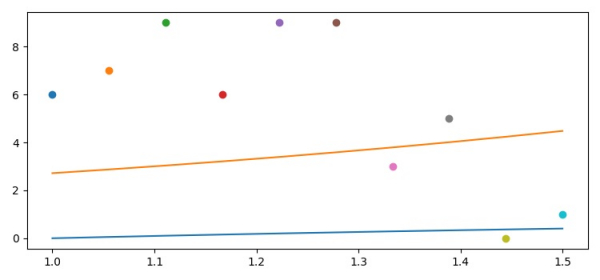# How to plot the lines first and points last in Matplotlib?

To plot the lines first and points last, we can take the following Steps −

• Create xpoints, y1points and y2points using numpy, to draw lines.

• Plot the curves using the plot() method with x, y1 and y2 points.

• Draw the scatter points using the scatter method.

• To display the figure, use the show() method.

## Example

import numpy as np
from matplotlib import pyplot as plt
plt.rcParams["figure.figsize"] = [7.50, 3.50]
plt.rcParams["figure.autolayout"] = True
xpoints = np.linspace(1, 1.5, 10)
y1points = np.log(xpoints)
y2points = np.exp(xpoints)
plt.plot(xpoints, y1points)
plt.plot(xpoints, y2points)
for i in xpoints:
plt.scatter(i, np.random.randint(10))
plt.show()

## Output